Next: The Essential Matrix Up: No Title Previous: Epipolar geometry examples

# Homogeneous Notation Interlude

Notation for plane (i.e. 2D) projective geometry. NB ``='' means equality up to scale.

• point: a pointis represented by the homogeneous 3-vector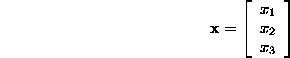where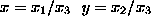and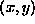are the inhomogeneous plane coordinates. Only the ratio of the homogeneous coordinates is significant.

• line: a lineis represented by the homogeneous 3-vector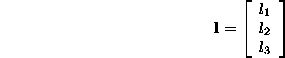for the line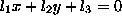. Again, only the ratio of the homogeneous line coordinates is significant.

• point on line: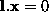or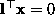or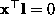• two points define a line: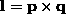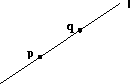• two lines define a point: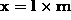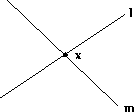• matrix notation for vector product: The vector product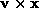can be represented as a matrix multiplication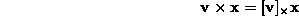where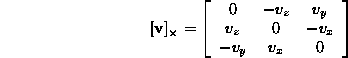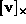is a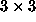skew-symmetric matrix of rank 2.is the one dimensional kernel of, since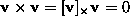.

Bob Fisher
Wed Apr 16 00:58:54 BST 1997